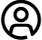# Logic Gates

Logic gates are physical components in a circuit that control the flow of a signal by implementing a boolean function, processing a signal to binary information. Logic gates are foundational in logic circuits, or in any electronic circuit process that involves digital to analogue signal conversion, or the flow of digital information.

The RS components range offers semiconductor logic gates from leading brands, with input/output configurations to suit the demands of your digital circuit.

Types of Logic Gate

Logic gates have distinct logic functions. The most common of these are known as basic gates. These include:

• AND Gates. This gate has multiple inputs that are processed to a single signal depending on the
positive combination of high signals in the inputs. It will only produce a high signal if both inputs register as high. (e.g two ones will result in a one, otherwise it will have an output of zero.)

• OR Gates. Like the AND gate, an OR gate has multiple inputs and a single output. In this case, it does not require a positive combination of one signals, but will implement logical produce a high signal if one or all of the inputs are high. (e.g a one and a zero, or two ones, may produce a high signal, but two zeros will produce zeros.)

• NOT Gates. Also known as inverters, the NOT gates will process the signal as the opposite of its value (eg. a one will invert as a zero, and a zero will invert as a 1.)

There are also combined function logic gates that modify typical functions with an inversion.

• NAND Gate. Unlike an AND gate, NAND will process two high signals as a zero and all other combinations as a one.

• NOR Gate. Unlike an OR gate, this will produce a one if the inputs register a zero signal in any combination, rather than a high signal.

• Buffer Gate. A buffer logic gate behaves in the opposite manner of a NOT gate, in that it passes a signal unchanged from input to output.

There are also logic gates referred to as Exclusive Gates.

• XNOR (EX-NOR) Gate. These gates process a high signal if all input signals are true, e.g: if there are two one signals or two zero signals.

• XOR Gate. Unlike an XNOR, XOR performs exclusive dis-junction, which means it will only output a true signal if exactly one of the inputs are true.

Applications

As CPUs employ binary logic, logic gates are essentially the building blocks of computers. As such they can be found in many kinds of digital circuits. Though their essential functions remain unchanged, their implementations are many.

• Encoders
• Decoders
• Multiplexers
• Demultiplexers
1411
Matches
Applied Filters
Filters
Display:
Results per page
20
• 20
• 50
• 100
 Price (Price shown excludes VAT) Part Details Brand Stock Logic Function Mounting Type Number Of Elements Number of Inputs per Gate Schmitt Trigger Input Package Type Pin Count Logic Family Input Type Maximum Operating Supply Voltage Maximum High Level Output Current Maximum Propagation Delay Time @ Maximum CL Minimum Operating Supply Voltage Maximum Low Level Output Current Output Type Propagation Delay Test Condition Dimensions Maximum Operating Temperature Length Automotive Standard Minimum Operating Temperature Height Width